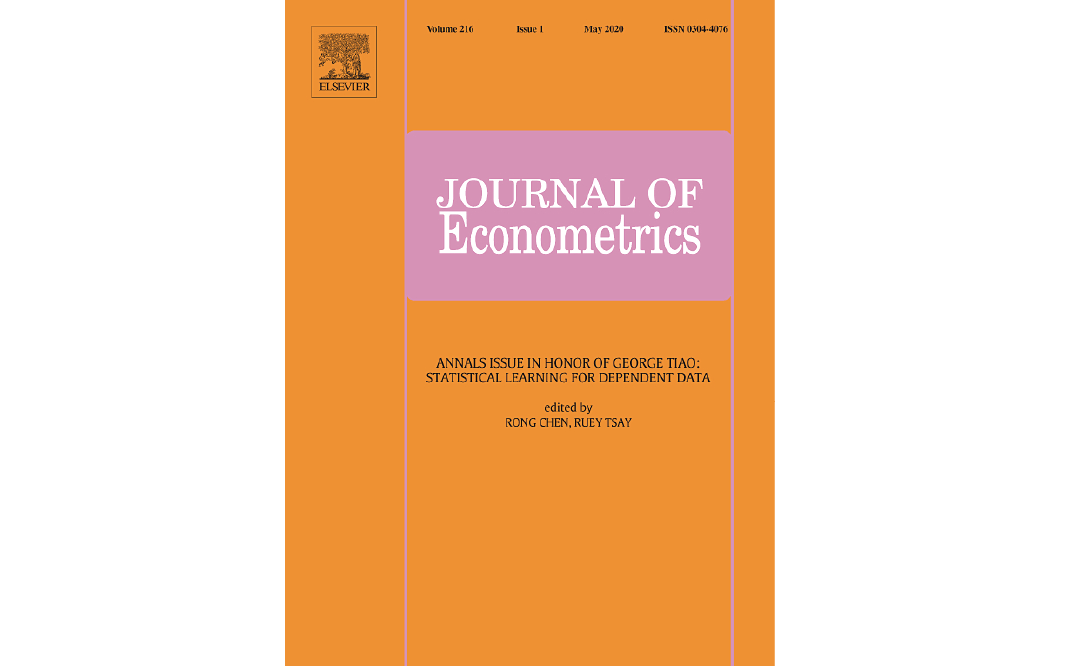• Estimation and Inference in a High-Dimensional Semiparametric Gaussian Copula Vector Autoregressive ModelYanqin Fan, Fang Han,  Hyeonseok Park   Published : November, 2023   JEL Code: C32, C51   URL to this Article: https://doi.org/10.1016/j.jeconom.2023.105513

Abstract

This paper develops simple, robust estimation and inference methods for the transition matrix of a high-dimensional semiparametric Gaussian copula vector autoregressive process. Our estimator is based on rank estimators of the large variance and auto-covariance matrices of a transformed latent high-dimensional Gaussian process. We derive rates of convergence of our estimator based on which we develop de-biased inference for Granger causality. Numerical results demonstrate the efficacy of the proposed methods. Although our focus is on the observable process, by the nature of rank estimators, all the methods developed directly apply to the transformed latent process. In technical terms, our analysis relies heavily on newly developed exponential inequalities for (degenerate) U-statistics under α-mixing condition.

Keywords

High-dimensional time series; Sparse transition matrix; α,-mixing; Latent Gaussian process; De-biasing inference; Kendall's tau